# Key Stage 3 Maths

In this assessment, students will review their comprehension of various topics across key stage 3. May take approximately 45 minutes to complete.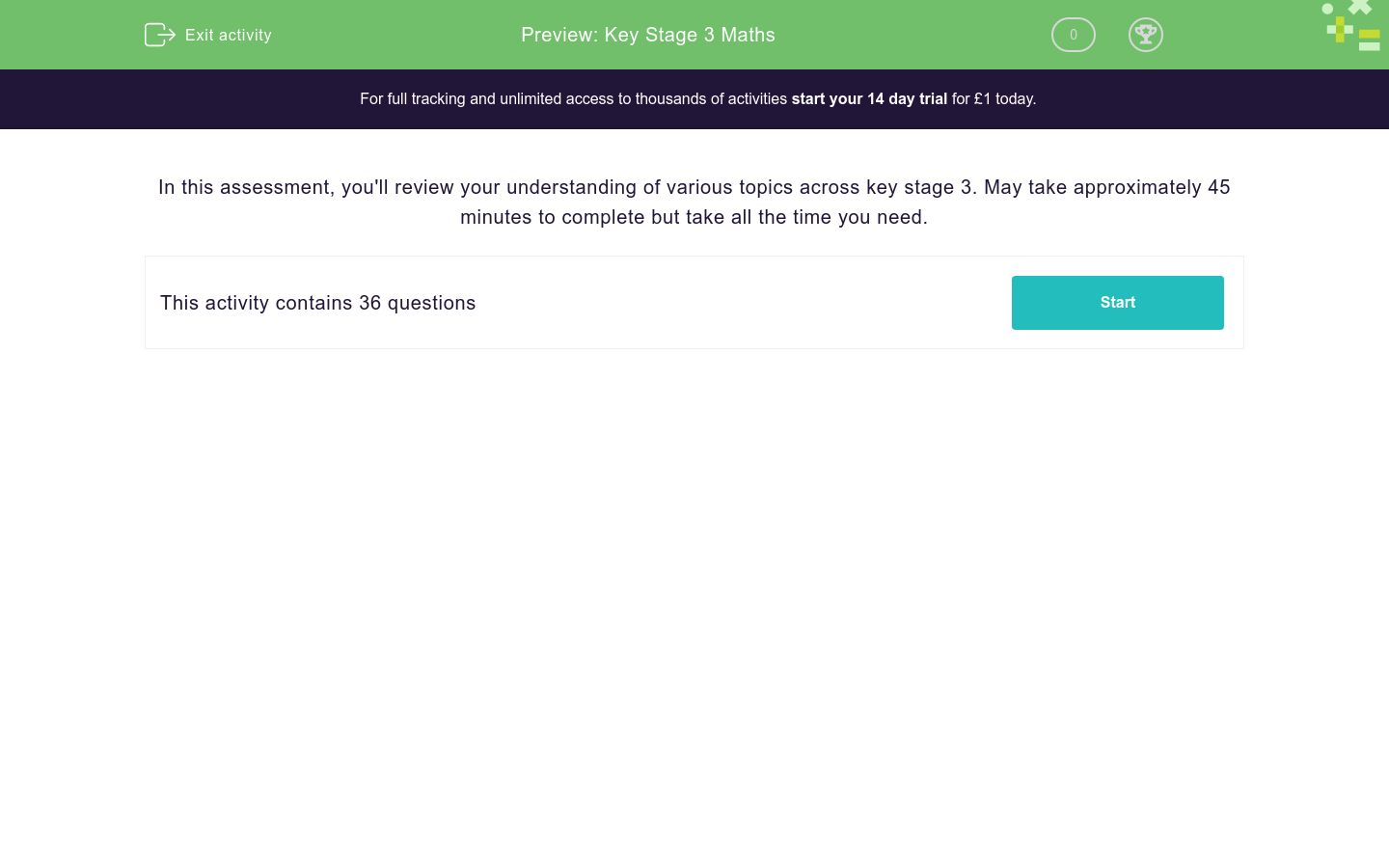Key stage:  KS 3

Curriculum topic:   End of Key Stage Assessments

Curriculum subtopic:   Starter Assessments

Difficulty level:### QUESTION 1 of 10

In this assessment, you'll review your understanding of various topics across key stage 3. May take approximately 45 minutes to complete but take all the time you need.

Work out:

840.3 + 8.403 + 84.03

Work out:

74.32 × 8.4

Work out:

78.39 × 8.7 ÷ 0.3

In a room of people:

½ of them wear glasses.

¼ of them wear contact lenses.

The rest have perfect eyesight.

What percentage have perfect eyesight?

(Just write the number.)

Which of these sequences will be the first to pass 1000?

A:    3     31     59     87 ...

B:    3     6     9     12 ...

C:    3     9     27     81 ...

A

B

C

By which of the given numbers is the following number divisible?

1 663 893

2

3

4

5

6

8

9

10

11

Round the following number to 2 decimal places.

42.259

Round the following number to 1, 2 and 3 significant figures.

37255

(Don't leave any space between the numbers.)

 answers 1 sig. fig. 2 sig. figs. 3 sig. figs.

Subtract these numbers, written in standard form

3.1 × 102  -  3.1 × 10-2

Work out:

 7 ÷ 5 40 20

(Give your answer as a fraction, reduced to its lowest terms, e.g. 3/10.)

Round each of these numbers to 1 sig. fig. to work out an approximate answer to:

 29.1 × 41.9 57.8You may use a calculator for this question.

At this year's annual fete, Jack sold 476 hotdogs, which is a 12% increase compared to last year.

How many hotdogs did he sell in total in the two years, i.e this year and last year?

Work out the nth term in this sequence:

4, 7, 10, 13, 16 ...

n + 3

3n + 1

4n + 3

4n + 7

Find the first 5 terms in the sequence given by the formula:

Tn = 5n2

where n is the position of the term in the sequence.

 value T1 T2 T3 T4 T5

Simplify:

a(-3a + 1) - 2a(a - 3)

-5a2 + 7a

-5a2 - 5a

-a2 - 5a

Solve the inequality:

6x + 4 ≤ -20

x < 3

x ≥ 4

x ≤ -4

x < -4

Form an equation from the angles shown in this triangle and solve it to find the value of a.

(Just write the number.)Select the correct HCF (Highest Common Factor) of these two algebraic expressions:

7p5q4 and  21pq6r6

7pq4r

14pq4r

21pq4r

Work out the value of the following expression when a = -16 and b = 72.

 a + b 2 8

Multiply out the two brackets and simplify:

(2x - 6)(4x + 6)

8x2 - 12x - 36

8x2 + 36x - 12

State the gradient of a line which is perpendicular to the line with equation:

y = 4 - 7x

(If it is not a whole number, write it as a fraction in the form a/b.)

A sum of money is shared in the ratio 5:3 between two people, A and B.

If A gets £95, how much does B get in £?

(Just write the number.)

A plane flies from London to Rome at an average speed of 668 km/h. The flying time is 2¼ hours.

Find the distance travelled by the plane in km.

(Just write the number.)

Convert the following area into one measured in cm2.

12.6 m2

(Just write the number.)

regular polygon has 8 sides.

Calculate its exterior angle.

(Just write the number.)You may use a calculator for this question.

Find the surface area of this cylinder in cm2 to 3 sig. figs.

(Just write the number.)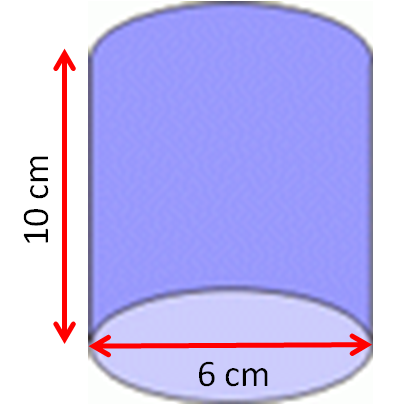You may use a calculator for this question.

The formula for the volume of a sphere, V, in terms of its radius, r, is:

V = 4/3πr3

sphere has a volume of 1000 cm3

Calculate its radius in cm and its surface area in cm2.

(Just write the number.)You may use a calculator for this question.

Use Pythagoras' Theorem to calculate the length of the hypotenuse, c, in the following right-angled triangle.You may use a calculator for this question.

Using trigonometry, calculate the side length x to 3 sig. figs.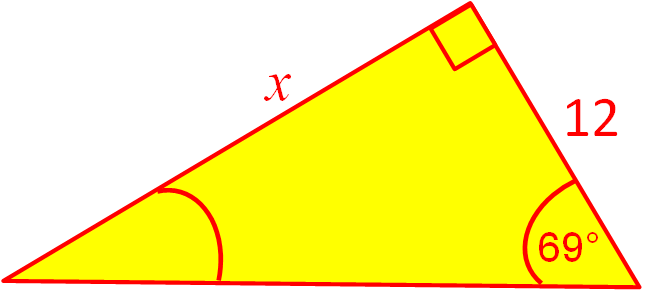You may use a calculator for this question.

Using trigonometry, find the height of this isosceles triangle in metres to 3 sig. figs.

(Just write the number.)One fair coin and one fair die are thrown.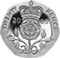Make a list of the possible outcomes on a piece of paper.

In how many outcomes is there a tail and an even number?

The tree diagram shows the probabilities that my bus is on time or late on Friday and Saturday.

Buses are usually late on Saturdays because it is market day.

Calculate the probability that it will be on time on one day and late on the other.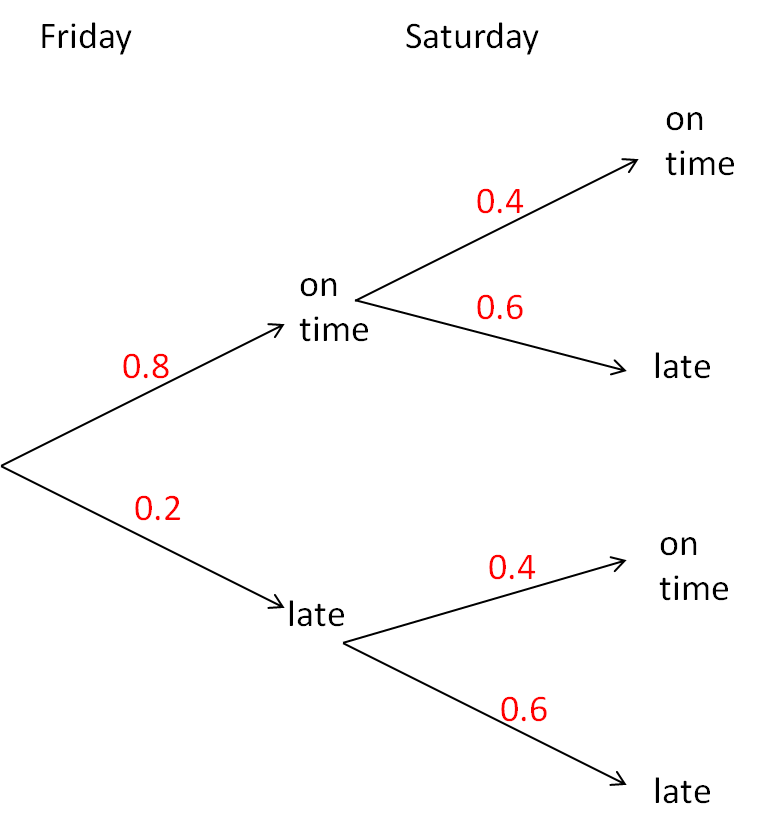These two fair spinners are spun and the two scores are added together.Use a sample-space diagram to answer the question.

What is the probability of getting a total score of 5?

(Write your answer with the / symbol, e.g. 2/3 and remember to reduce your fraction to its lowest terms.)

Which line is the line of best fit on this scatter graph?A

B

C

This chart shows the heights of children in a Year 9 class at school.

Find the median class.110 cm to 115 cm

115 cm to 120 cm

120 cm to 125 cm

125 cm to 130 cm

130 cm to 135 cm

135 cm to 140 cm

140 cm to 145 cm

145 cm to 150 cm

150 cm to 155 cmYou may use a calculator for this question.

This table shows the masses of children in a Year 9 class at school.

Estimate the total mass in kg of all the children in the class.

Mass (m kg) Frequency
40 ≤ m < 45 2
45 ≤ m < 50 5
50 ≤ m < 55 9
55 ≤ m < 60 3
60 ≤ m < 65 1
• Question 1

Work out:

840.3 + 8.403 + 84.03

932.733
EDDIE SAYS
Line up the decimal points, and add the digits in columns.
• Question 2

Work out:

74.32 × 8.4

624.288
EDDIE SAYS
Use long multiplication to work out 7432 × 84.
Then divide the answer by 1000.
• Question 3

Work out:

78.39 × 8.7 ÷ 0.3

2273.31
EDDIE SAYS
Use long multiplication to work out 7839 × 87.
Then divide the answer by 1000 to get 681.993.
Then multiply both numbers by 10 to give 6819.93 ÷ 3.
• Question 4

In a room of people:

½ of them wear glasses.

¼ of them wear contact lenses.

The rest have perfect eyesight.

What percentage have perfect eyesight?

(Just write the number.)

25
EDDIE SAYS
¼ of the people in the room have perfect eyesight.
¼ of 100 = 25.
• Question 5

Which of these sequences will be the first to pass 1000?

A:    3     31     59     87 ...

B:    3     6     9     12 ...

C:    3     9     27     81 ...

C
EDDIE SAYS
C shows the powers of 3, which increase very quickly.
The next number is 243, then 729.
The next number will be more than 1000.
• Question 6

By which of the given numbers is the following number divisible?

1 663 893

3
9
11
EDDIE SAYS
2: ends in odd digit.
3: digit sum is 36, which is divisible by 3.
4: last two-digit number is not divisible by 4.
5: does not end in 0 or 5.
6: not divisible by 2.
8: last three-digit number is not divisible by 8.
9: digit sum is 36, which is divisible by 9.
10: does not end in 0.
11: compare alternate digit sums which are both 18.
• Question 7

Round the following number to 2 decimal places.

42.259

42.26
EDDIE SAYS
42.259 is between 42.25 and 42.26, but it is nearer to 42.26.
• Question 8

Round the following number to 1, 2 and 3 significant figures.

37255

(Don't leave any space between the numbers.)

 answers 1 sig. fig. 2 sig. figs. 3 sig. figs.
EDDIE SAYS
1 sig. fig: 37255 is between 30000 and 40000 but nearer to 40000.
2 sig. figs: 37255 is between 37000 and 38000 but nearer to 37000.
3 sig. figs: 37255 is between 37200 and 37300 but nearer to 37300.
• Question 9

Subtract these numbers, written in standard form

3.1 × 102  -  3.1 × 10-2

309.969
EDDIE SAYS
3.1 × 102 = 310
3.1 × 10-2 = 0.031
310 - 0.031 = 309.969
• Question 10

Work out:

 7 ÷ 5 40 20

(Give your answer as a fraction, reduced to its lowest terms, e.g. 3/10.)

7/10
EDDIE SAYS
Turn second fraction upside down and multiply.
7/40 × 20/5
Reduce to get 7/10.
• Question 11

Round each of these numbers to 1 sig. fig. to work out an approximate answer to:

 29.1 × 41.9 57.8
20
EDDIE SAYS
30 × 40 = 1200
1200 ÷ 60 = 20
• Question 12You may use a calculator for this question.

At this year's annual fete, Jack sold 476 hotdogs, which is a 12% increase compared to last year.

How many hotdogs did he sell in total in the two years, i.e this year and last year?

901
EDDIE SAYS
476 ÷ 1.12 = 425
425 + 476 = 901
• Question 13

Work out the nth term in this sequence:

4, 7, 10, 13, 16 ...

3n + 1
EDDIE SAYS
Common difference is 3.
• Question 14

Find the first 5 terms in the sequence given by the formula:

Tn = 5n2

where n is the position of the term in the sequence.

 value T1 T2 T3 T4 T5
EDDIE SAYS
T1 = 5 × 12 = 5 × 1 = 5
T2 = 5 × 22 = 5 × 4 = 20
T3 = 5 × 32 = 5 × 9 = 45
T4 = 5 × 42 = 5 × 16 = 80
T5 = 5 × 52 = 5 × 25 = 125
• Question 15

Simplify:

a(-3a + 1) - 2a(a - 3)

-5a2 + 7a
EDDIE SAYS
-3a2 + a - 2a2 + 6a
• Question 16

Solve the inequality:

6x + 4 ≤ -20

x ≤ -4
EDDIE SAYS
6x ≤ -24
• Question 17

Form an equation from the angles shown in this triangle and solve it to find the value of a.

(Just write the number.)20
EDDIE SAYS
60 - a + 3a + 30 + 4a - 30 = 180
60 + 6a = 180
6a = 120
a = 20
• Question 18

Select the correct HCF (Highest Common Factor) of these two algebraic expressions:

7p5q4 and  21pq6r6

7pq4r
EDDIE SAYS
The HCF of 7 and 21 is 7.
Choose the smaller of the powers of each letter.
• Question 19

Work out the value of the following expression when a = -16 and b = 72.

 a + b 2 8
1
EDDIE SAYS
-8 + 9
• Question 20

Multiply out the two brackets and simplify:

(2x - 6)(4x + 6)

8x2 - 12x - 36
EDDIE SAYS
2x(4x + 6) - 6(4x + 6) = 8x2 + 12x - 24x - 36
• Question 21

State the gradient of a line which is perpendicular to the line with equation:

y = 4 - 7x

(If it is not a whole number, write it as a fraction in the form a/b.)

1/7
EDDIE SAYS
The gradient of the given line is -7.
A perpendicular line will have gradient 1/7, so that the gradients of the two lines multiply to give -1.
• Question 22

A sum of money is shared in the ratio 5:3 between two people, A and B.

If A gets £95, how much does B get in £?

(Just write the number.)

57
EDDIE SAYS
There are £95 in 5 bags, so each bag contains £95 ÷ 5 = £19.
B gets 3 bags, i.e. 3 × £19 = £57.
• Question 23

A plane flies from London to Rome at an average speed of 668 km/h. The flying time is 2¼ hours.

Find the distance travelled by the plane in km.

(Just write the number.)

1503
EDDIE SAYS
668 × 2¼ = 668 + 668 + 668 ÷ 4 = 668 + 668 + 167
• Question 24

Convert the following area into one measured in cm2.

12.6 m2

(Just write the number.)

126000
126 000
EDDIE SAYS
There are 100 × 100 = 10 000 cm2 in 1 m2.
• Question 25

regular polygon has 8 sides.

Calculate its exterior angle.

(Just write the number.)

45
EDDIE SAYS
360 ÷ 8
• Question 26You may use a calculator for this question.

Find the surface area of this cylinder in cm2 to 3 sig. figs.

(Just write the number.)245
EDDIE SAYS
Two circles and a rectangle.
The base of the rectangle is the circumference of the circle.
2 × π × 3² + 6 × π × 10
• Question 27You may use a calculator for this question.

The formula for the volume of a sphere, V, in terms of its radius, r, is:

V = 4/3πr3

sphere has a volume of 1000 cm3

Calculate its radius in cm and its surface area in cm2.

(Just write the number.)

EDDIE SAYS
Radius = ³√ [(3 × 1000) ÷ (4 × π)]
Surface area = 4 × π × r2
Do not round off the radius to find the surface area.
• Question 28You may use a calculator for this question.

Use Pythagoras' Theorem to calculate the length of the hypotenuse, c, in the following right-angled triangle.10.4
EDDIE SAYS
Work out 32 + 102 = 109.
√109 = 10.440...
• Question 29You may use a calculator for this question.

Using trigonometry, calculate the side length x to 3 sig. figs.31.3
EDDIE SAYS
x = 12 × tan69°
• Question 30You may use a calculator for this question.

Using trigonometry, find the height of this isosceles triangle in metres to 3 sig. figs.

(Just write the number.)13.4
EDDIE SAYS
height = 2.6/tan11º
• Question 31

One fair coin and one fair die are thrown.Make a list of the possible outcomes on a piece of paper.

In how many outcomes is there a tail and an even number?

3
EDDIE SAYS
T2, T4, T6
• Question 32

The tree diagram shows the probabilities that my bus is on time or late on Friday and Saturday.

Buses are usually late on Saturdays because it is market day.

Calculate the probability that it will be on time on one day and late on the other.0.56
EDDIE SAYS
0.8 × 0.6 + 0.2 × 0.4 = 0.48 + 0.08 = 0.56
• Question 33

These two fair spinners are spun and the two scores are added together.Use a sample-space diagram to answer the question.

What is the probability of getting a total score of 5?

(Write your answer with the / symbol, e.g. 2/3 and remember to reduce your fraction to its lowest terms.)

1/4
EDDIE SAYS
1 2 3 4
1 2 3 4 5
2 3 4 5 6
3 4 5 6 7
4 5 6 7 8

The probability of getting a total of 5, P(5) = 4/16 = 1/4.

• Question 34

Which line is the line of best fit on this scatter graph?C
EDDIE SAYS
The line C goes through the most points and follows the general direction of the points.
• Question 35

This chart shows the heights of children in a Year 9 class at school.

Find the median class.130 cm to 135 cm
EDDIE SAYS
No. of children in class = 1 + 3 + 7 + 5 + 4 + 3 + 1 = 24
We need to find the 12th and 13th child in order of height.
They will both be in the 130 cm to 135 cm group, because the first 11 children are in the first 3 groups shown.
• Question 36You may use a calculator for this question.

This table shows the masses of children in a Year 9 class at school.

Estimate the total mass in kg of all the children in the class.

Mass (m kg) Frequency
40 ≤ m < 45 2
45 ≤ m < 50 5
50 ≤ m < 55 9
55 ≤ m < 60 3
60 ≤ m < 65 1
1030
EDDIE SAYS
Total is (2 × 42.5) + (5 × 47.5) + (9 × 52.5) + (3 × 57.5) + (1 × 62.5) = 1030 kg
---- OR ----

Sign up for a £1 trial so you can track and measure your child's progress on this activity.

### What is EdPlace?

We're your National Curriculum aligned online education content provider helping each child succeed in English, maths and science from year 1 to GCSE. With an EdPlace account you’ll be able to track and measure progress, helping each child achieve their best. We build confidence and attainment by personalising each child’s learning at a level that suits them.

Get started